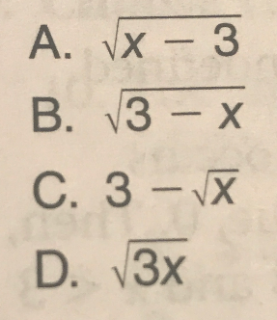## Labels

### GED Math Review: Undefined Expressions

Questions:
Directions: Choose or write the answer to each question.

1. Which of the following is all the values of x for whichis undefined?
• x = 0
• x = 2
• x = -2 , x = 0, or x = 2
• x = -2 or x = 2

2. Which of the following is all values of x for whichis undefined?
• x < 0
• x = -5 or x = 5
• -5 < x < 5
• x > 5 or x < -5
3. Which of the following is undefined for all values of x such that x < 3?4. Which of the following is undefined for all values of x such ≥ 2?5. Which of the following is all the values of x for whichis undefined?
• x = -4 or x = 4
• x < 0
• -4 ≤ x ≤ -4
• x ≥ 4 or ≤ -4

For questions 7 and 8, three choices for values of x are given. In the box beside each expression, write the choice for which each expressions is undefined.

1. x = -2 or x = 2
2. x > 5 or x < -5
3. C
4. A
5. B
6. x ≥ 4 or ≤ -4
7. I (x = -2 or x = 0)
II x = -2
III x = 2
8. I -6 < x < 6
II x < 6
III x > 6 or x < -6

Reference:

Complete Test Preparation for the GED Test 2014 by Steck-Vaughn